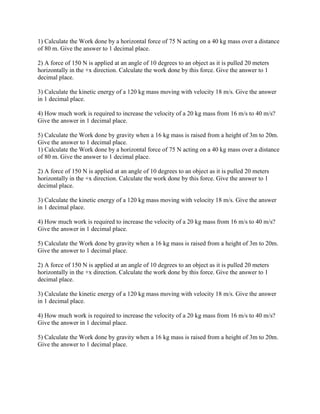Diese Präsentation wurde erfolgreich gemeldet.

# 1) Calculate the Work done by a horizontal force of 75 N acting on a 4.docx

Anzeige
Anzeige
Anzeige
Anzeige
Anzeige
Anzeige
Anzeige
Anzeige
Anzeige
Anzeige
Anzeige×

1 von 2 Anzeige

# 1) Calculate the Work done by a horizontal force of 75 N acting on a 4.docx

1) Calculate the Work done by a horizontal force of 75 N acting on a 40 kg mass over a distance of 80 m. Give the answer to 1 decimal place.

2) A force of 150 N is applied at an angle of 10 degrees to an object as it is pulled 20 meters horizontally in the +x direction. Calculate the work done by this force. Give the answer to 1 decimal place.

3) Calculate the kinetic energy of a 120 kg mass moving with velocity 18 m/s. Give the answer in 1 decimal place.

4) How much work is required to increase the velocity of a 20 kg mass from 16 m/s to 40 m/s? Give the answer in 1 decimal place.

5) Calculate the Work done by gravity when a 16 kg mass is raised from a height of 3m to 20m. Give the answer to 1 decimal place.
1) Calculate the Work done by a horizontal force of 75 N acting on a 40 kg mass over a distance of 80 m. Give the answer to 1 decimal place.

2) A force of 150 N is applied at an angle of 10 degrees to an object as it is pulled 20 meters horizontally in the +x direction. Calculate the work done by this force. Give the answer to 1 decimal place.

3) Calculate the kinetic energy of a 120 kg mass moving with velocity 18 m/s. Give the answer in 1 decimal place.

4) How much work is required to increase the velocity of a 20 kg mass from 16 m/s to 40 m/s? Give the answer in 1 decimal place.

5) Calculate the Work done by gravity when a 16 kg mass is raised from a height of 3m to 20m. Give the answer to 1 decimal place.

2) A force of 150 N is applied at an angle of 10 degrees to an object as it is pulled 20 meters horizontally in the +x direction. Calculate the work done by this force. Give the answer to 1 decimal place.

3) Calculate the kinetic energy of a 120 kg mass moving with velocity 18 m/s. Give the answer in 1 decimal place.

4) How much work is required to increase the velocity of a 20 kg mass from 16 m/s to 40 m/s? Give the answer in 1 decimal place.

5) Calculate the Work done by gravity when a 16 kg mass is raised from a height of 3m to 20m. Give the answer to 1 decimal place.
Solution
1) work = force*dispalcement
w = 75*80 = 6*10^3 J
2) w = fscos(theta)
w = 150*20*cos10 = 2.9*10^3 J
3) k = 1/2mv^2 = 1/2*120*18^2 = 1.9*10^4 J
4) w = 1/2*20(40^2-16^2) = 1.3*10^4 J
5) w = 16*9.8(20-3) = 2.7*10^3 J
.

1) Calculate the Work done by a horizontal force of 75 N acting on a 40 kg mass over a distance of 80 m. Give the answer to 1 decimal place.

2) A force of 150 N is applied at an angle of 10 degrees to an object as it is pulled 20 meters horizontally in the +x direction. Calculate the work done by this force. Give the answer to 1 decimal place.

3) Calculate the kinetic energy of a 120 kg mass moving with velocity 18 m/s. Give the answer in 1 decimal place.

4) How much work is required to increase the velocity of a 20 kg mass from 16 m/s to 40 m/s? Give the answer in 1 decimal place.

5) Calculate the Work done by gravity when a 16 kg mass is raised from a height of 3m to 20m. Give the answer to 1 decimal place.
1) Calculate the Work done by a horizontal force of 75 N acting on a 40 kg mass over a distance of 80 m. Give the answer to 1 decimal place.

2) A force of 150 N is applied at an angle of 10 degrees to an object as it is pulled 20 meters horizontally in the +x direction. Calculate the work done by this force. Give the answer to 1 decimal place.

3) Calculate the kinetic energy of a 120 kg mass moving with velocity 18 m/s. Give the answer in 1 decimal place.

4) How much work is required to increase the velocity of a 20 kg mass from 16 m/s to 40 m/s? Give the answer in 1 decimal place.

5) Calculate the Work done by gravity when a 16 kg mass is raised from a height of 3m to 20m. Give the answer to 1 decimal place.

2) A force of 150 N is applied at an angle of 10 degrees to an object as it is pulled 20 meters horizontally in the +x direction. Calculate the work done by this force. Give the answer to 1 decimal place.

3) Calculate the kinetic energy of a 120 kg mass moving with velocity 18 m/s. Give the answer in 1 decimal place.

4) How much work is required to increase the velocity of a 20 kg mass from 16 m/s to 40 m/s? Give the answer in 1 decimal place.

5) Calculate the Work done by gravity when a 16 kg mass is raised from a height of 3m to 20m. Give the answer to 1 decimal place.
Solution
1) work = force*dispalcement
w = 75*80 = 6*10^3 J
2) w = fscos(theta)
w = 150*20*cos10 = 2.9*10^3 J
3) k = 1/2mv^2 = 1/2*120*18^2 = 1.9*10^4 J
4) w = 1/2*20(40^2-16^2) = 1.3*10^4 J
5) w = 16*9.8(20-3) = 2.7*10^3 J
.

Anzeige
Anzeige